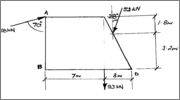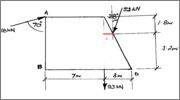Determine the moment about a given point

Homework Statement

I'm trying to find the moments about points A,B and D respectively.Mx= F * d

The Attempt at a Solution

Correct me if I'm wrong, but on point A the leftmost force produces no moment. After that I'm a little confused about what to use for the distances of the other two forces.

PhanthomJay
Homework Helper
Gold Member

Homework Statement

I'm trying to find the moments about points A,B and D respectively.Mx= F * d

The Attempt at a Solution

Correct me if I'm wrong, but on point A the leftmost force produces no moment.
That is correct.
After that I'm a little confused about what to use for the distances of the other two forces.
Breakup the 52 kN force into its vertical and horizontal vector components, applied where the arrow of the 52 kN force is is touching the line. Then remember the golden rule: The moment of a force, or component of that force, is equal to the force, or component of that force, times the perpendicular distance from the line of action of the force, or component of that force, to the point about which you are summing moments.
For example, looking now at the 93 kN force, its line of action is a vertical line. The moment of that force about A is then 93*(___?__), clockwise. Now calculate the moments for each of the force components of the 52 kN force, and add them all up.

you may also need to find the angle in the triangle made after a square.

sorry rectangle

so I can use 1.8m as the vertical distance, but by the way it looks I guess I have to find out the length of x and add it to 7 in order to find the horizontal distance.For 52 sin 28 its 1.8 m and for 52 cos 28 you have to find the angle to find the distance which should be added with 7 m

nvn